# The determinant of a 2 x 2 matrix

### The determinant of a 2 x 2 matrix

In this lesson, we will learn how to find a determinant of a 2 x 2 matrix. To find the determinant, we multiply the top left entry and bottom right entry and subtract it with the product of the top right entry and bottom left entry. We will use these determinants later on in the course to show if a matrix is invertible. We will also use it to find inverses of 2 x 2 matrices.
Basic Concepts: Notation of matrices

#### Lessons

Let the matrix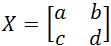, where $a, b, c,$ and $d$ are all numbers. We denote the determinant of $X$ to be det$(X)$. To find the determinant of a 2 x 2 matrix we compute the following:

det$(X)=ad-bc$
• Introduction
The determinant of a 2 x 2 matrix Overview

• 1.
Finding the Determinant
You are given that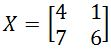. Find the determinant.

• 2.
You are given that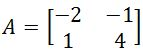. Find the determinant.

• 3.
You are given that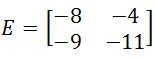. Find the determinant.

• 4.
You are given that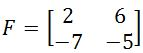. Find the determinant.

• 5.
You are given that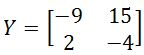. Find the determinant.

• 6.
You are given that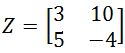. Find the determinant.